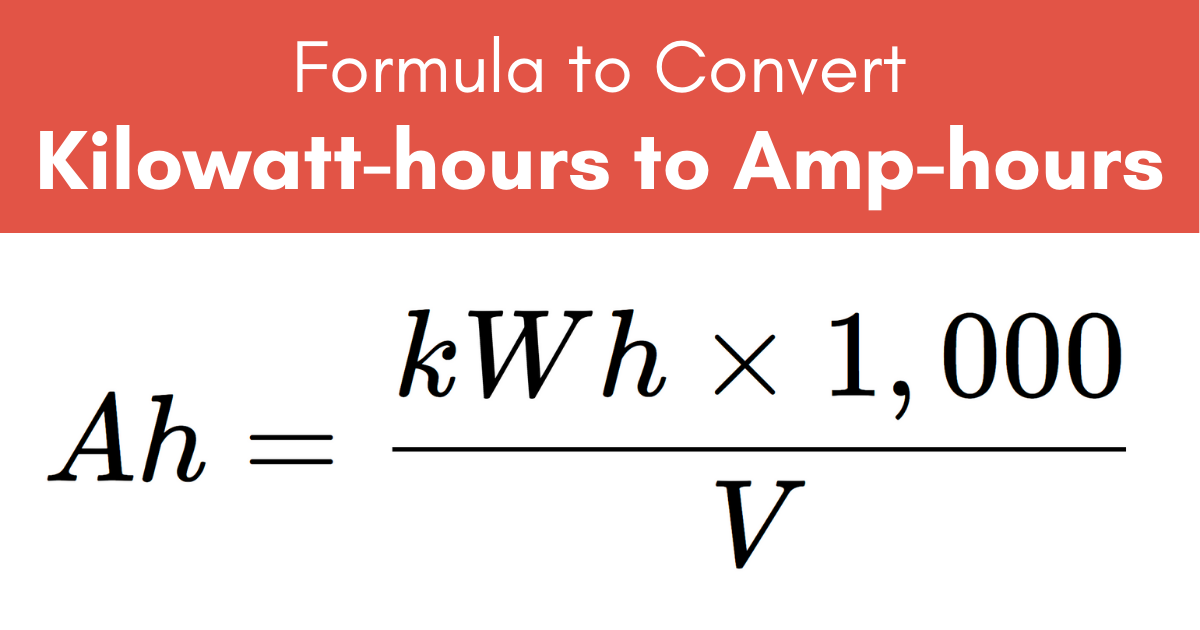# Kilowatt-Hours (kWh) to Amp-Hours (Ah) Conversion Calculator

Convert amp-hours to kilowatt-hours using the calculator below by entering the electrical charge in Ah along with the voltage.

kWh
V

## Amp-Hours Results:

Ah
Learn how we calculated this below

Do you want to convert Ah to kWh?

## How to Convert Kilowatt-Hours to Amp-Hours

Kilowatt-hours, abbreviated kWh or kW·h, are a measure of electrical energy. The energy equal to one kWh is equal to one kilowatt, or one thousand watts of power, consumed for one hour of time.

Amp-hours, abbreviated Ah or A·h, are a measure of electrical charge, and are often used to measure the charge capacity of batteries. One Ah is the amount of electrical charge transferred by one amp of current in one hour of time.

You can convert kilowatt-hours to amp-hours using a formula.

### kWh to Ah Conversion Formula

Converting from electrical energy to electrical charge can be done using the formula below. The voltage at which the charge is held must also be known.

Ah = kWh × 1,000 / V

The electrical charge in amp-hours is equal to the energy in kilowatt-hours times 1,000, then divided by the voltage.For example, let’s convert 5 kWh at 120 V to Ah.

Ah = 5 kWh × 1,000 / 120 V
Ah = 5,000 Wh / 120 V
Ah = 41.667 Ah

So, in this example, 5 kWh is equal to 41.667 Ah.

You might also want to convert watt-hours to milliamp-hours.